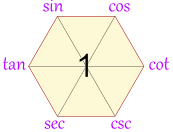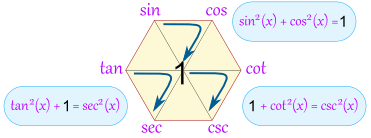# Basics of Trigonometry

Trigonometry (from Greek trigōnon, “triangle” and metron, “measure”) is a branch of mathematics that studies relationships between side lengths and angles of triangles.

A triangle has three sides and three angles.

The three angles always add to 180°.

There are three special names given to triangles that tell how many sides (or angles) are equal.Note: In Formulae and Tables booklet lengths of sides of shapes are designated by lower case (small) letters, and angles are designated by upper case (capital) letters.When a triangle has a right angle (90°) …

… and squares are made on each of the three sides, ..

… then the biggest square has the exact same area as the other two squares put together!The longest side of the triangle is called the “hypotenuse”, so the formal definition is:

In a right angled triangle:
the square of the hypotenuse is equal to
the sum of the squares of the other two sides.

If we know the lengths of two sides of a right angled triangle, we can find the length of the third side. (But remember it only works on right angled triangles!)

Trigonometric functions relate an angle of a right-angled triangle to ratios of two side lengths.SOHCAHTOAIf you have two triangles in which the angles in one are the same as the angles in the other, then the ratio of corresponding sides will be the same for each triangle.Check out this site for nice simple and clear descriptions of different aspects of Trigonometry.    http://www.mathsisfun.com/algebra/trigonometry-index.html

including the piece below on the Magic Hexagon.

# Magic Hexagon for Trig Identities

 This hexagon is a special diagram to help you remember many trigonometric identities## Building It: The Quotient Identities

 Start with:tan(x) = sin(x) / cos(x)(This one you just have to remember)Then add: cot (which is cotangent) opposite tan csc (which is cosecant) next, and sec (which is secant) lastTo help you remember: the “co” functions are all on the right

OK, we have now built our hexagon, what do we get out of it?

Well, we can now follow “around the clock” (either direction) to get all the “Quotient Identities”:

 Clockwise Anticlockwise tan(x) = sin(x) / cos(x) sin(x) = cos(x) / cot(x) cos(x) = cot(x) / csc(x) cot(x) = csc(x) / sec(x) csc(x) = sec(x) / tan(x) sec(x) = tan(x) / sin(x) cos(x) = sin(x) / tan(x) sin(x) = tan(x) / sec(x) tan(x) = sec(x) / csc(x) sec(x) = csc(x) / cot(x) csc(x) = cot(x) / cos(x) cot(x) = cos(x) / sin(x)

## Product Identities

The hexagon also shows that a function between any two functions is equal to them multiplied together (if they are opposite each other, then the “1” is between them):Example: tan(x)cos(x) = sin(x) Example: tan(x)cot(x) = 1

Some more examples:

• sin(x)csc(x) = 1
• tan(x)csc(x) = sec(x)
• sin(x)sec(x) = tan(x)

## But Wait, There is More!

You can also get the “Reciprocal Identities”, by going “through the 1”Here you can see that sin(x) = 1 / csc(x)

Here is the full set:

• sin(x) = 1 / csc(x)
• cos(x) = 1 / sec(x)
• cot(x) = 1 / tan(x)
• csc(x) = 1 / sin(x)
• sec(x) = 1 / cos(x)
• tan(x) = 1 / cot(x)

## Bonus!

AND we also get these:Examples: sin(30°) = cos(60°) tan(80°) = cot(10°) sec(40°) = csc(50°)

## Double Bonus: The Pythagorean Identities

The Unit Circle shows us that

sin2 x + cos2 x = 1

The magic hexagon can help us remember that, too, by going around any of these three triangles:And we have: sin2(x) + cos2(x) = 1 1 + cot2(x) = csc2(x) tan2(x) + 1 = sec2(x)

You can also travel backwards around a triangle. For example:

• 1 – cos2(x) = sin2(x)

Trigonometry Tutorial from Engineers Ireland for Leaving Cert Maths. Lasts an hour and three quarters but you can always look at it in smaller bits at a time.

Trigonometry is one of the most important topics in Higher Level Leaving Cert maths. It is hard to avoid trigonometry, as well as accounting for questions in its own right, trigonometry is required in many other areas of the course, e.g., complex numbers, differentiation, integration and vectors.

Originally trigonometry was purely the study of triangles, but it has been expanded greatly since then. Our course reflects this development. We study triangles, sectors, circles, trigonometry ratios as well as the more general trigonometry functions. We learn how to prove trigonometry identities and how to solve trigonometry equations. We also examine inverse trigonometry functions and investigate a well-known trigonometry limit.

# TRIGONOMETRIC PROOFS – HINTS AND GUIDELINES

Trigonometric Identity Proving would often cause even the top secondary school students to break out in cold sweat. This is because  trigonometry proving questions do not have a standard “plug and play” method of solving. Every question is a new puzzle for which the students have to find a route from start to finish.

Although each question is unique, there are several “rules of thumb” which students can follow. Here are some tips to help students conquer Trigonometric proving.

## General Approach

You should be familiar with the various trigonometric identities, like the reciprocal trigonometric functions and the Pythagorean identities, which can be found in your Formulae and Tables booklet.

There are many different ways to prove an identity. There are multiple correct answers to almost every identity. If it takes you 10 steps, and someone else did it differently in 5 steps, and both use correct transformations, then both of you are 100% correct!

1. Work on the side that is more complicated. Try and simplify it. It is easier to eliminate terms to make a complex function simple than to find ways to introduce terms to make a simple function complex.

2. Replace all trigonometric functions with just sinθ and cosθ where possible. This is to standardize both sides of the trigonometric identity so that it is easier to compare one side to another.

3. Identify algebraic operations like factoring, expanding, distributive property, adding and multiplying fractions. Add and combine fractions to a single fraction; (get common denominators). This allows us to simplify the expression further. Square Binomials. Remember which functions pair up well (especially when squared): Sine and Cosine, Tangent and Secant, Cotangent and Cosecant.

4. Use the various trigonometric identities given in the formulae booklet. In particular, watch out for the Pythagorean identity. Pay special attention to addition of squared trigonometry terms. Apply the Pythagorean identities when necessary. Especially sin²x+cos²x=1 if all the other trig terms have been converted into sine and cosine. This identity can be used to convert into and vice versa. It can also be used to remove both by turning it into 1.

5. Know when to Apply Double Angle Formula (DAF). Observe every trigonometric term in the question. Are there terms with angles that are 2 times of another? If there are, be ready to use DAF to transform them into the same angle. For example, if you see sinθ and cot(θ/2) in the same question, you have to use DAF since θ is 2 times of (θ/2).

6. Keep an eye on the other side, and work towards it. If you get stuck, try working from the other side. You might end up in the middle. If you do, then you can just re-write that part in reverse order.

7. Consider the “trigonometric conjugate.”

8. When Desperate… Pretend! Disclaimer: Only use this tactic if you find yourself stuck half way during the trigonometric proving process in an examination (with the clock running down) and you do not want to jeopardize the rest of the paper. Since you are stuck mid way, simply complete the question by pretending that you have proved the identity. From your current step, jump straight to the final step and then write (=RHS (Proven)). After the exam, remember to pray that the marker is either blind or compassionate enough to give you the benefit of the doubt and award you the marks. And seen as the examiner is likely to be correcting many scripts they just might.

9. Practice! Practice! Practice! trigonometric function proving becomes relatively easy after you have conquered a massive number questions and exposed yourself to all the different varieties of questions. There are no hard and fast rule to handling trigonometry proving questions since every question is like a puzzle. But once you have solved a puzzle before, it becomes easier to solve the same puzzle again.

10. Do not try to Prove a Question that says “Solve”! After practicing a lot of proving questions, some students develop a robotic tendency to prove LHS = RHS whenever they see an equation with trigonometric functions. Even when they encounter a question that says “Solve the trigonometry equation…”… Do read the question carefully! If the question expects you to “Solve”, do not try to prove it! Example: Solve the equation 5 cosecx – 3 sinx = 5 cotx. This is a “solve question” (i.e. find the values of x ). DO NOT attempt to prove it because you can’t!  This is why teachers keep repeating read the question fully and answer the question you are asked not the one you want to be asked.